# Alphabet (Part 1) CLAT Notes | EduRev

## CLAT : Alphabet (Part 1) CLAT Notes | EduRev

The document Alphabet (Part 1) CLAT Notes | EduRev is a part of the CLAT Course Logical Reasoning for CLAT.
All you need of CLAT at this link: CLAT

CHAPTER - 1

ENGLISH ALPHABET

All the test papers of reasoning in the competitive examinations start with a question based on ‘Alphabet’. Depending on the type and selection criteria, the numbers of questions vary. The understanding of English Alphabet is also essential to solve questions found in Coding – Decoding, Classification and Series.

To solve the questions based on alphabets one should remember the position of alphabet from either side. Usually a number of words are given in the series which individually are called the terms and there is a definite relationship between each term. The candidate should be able to recall the position of the alphabet fast enough to solve these problems and know the position not only from left but from right as well.

CODE BASED ON ENGLISH ALPHABET

In order to solve questions pertaining to code based on English alphabet, you must know the position of each letter from either end so that you can recognize the coding pattern quickly.

POSITION OF ALPHABETS FROM LEFT TO RIGHT:

 1 2 3 4 5 6 7 8 9 10 11 12 13 A B C D E F G H I J K L M

 14 15 16 17 18 19 20 21 22 23 24 25 26 N O P Q R S T U V W X Y Z

To memorise the position from left, the student should first learn the EJOTY PRINICIPLE as explained here under: Each letter in the term EJOTY represents the position, which is multiple of 5. Thus,

 A F K P U B G L Q V C H M R W D I N S X E J O T Y 5th 10th 15th 20th 25th

Based on knowledge of EJOTY, where E represents 5th position of alphabet , J is 10th , O is 15th, T is 20th and Y is 25th , one call easily find out the position of all other alphabets. But the final key is recalling the position of each alphabet without even referring to EJOTY.

TO SOLVE QUESTION BASED ON ALPHABETS, USE FOLLOWING RULES:

RULE 1:TO FIND THE POSITION OF ALPHABET FROM L.H.S / R.H.S:

If the position of an alphabet from left is known and we are required to find out its position from right, then just subtract the given position from 27 and we get its position of alphabet from right.

Directions (1-5): In the alphabet series we are given the position of alphabet from L.H.S.  What is the position of alphabet from R.H.S.?

Ex 1: J is 10th alphabet from left.
Ex 2: H is 8th alphabet from left.
Ex 3: T is 20th alphabet from left.
Ex 4: L is 12th alphabet from left.
Ex 5: X is 24th alphabet from left

Ans 1: 27 – 10 =  17th from RHS.
Ans 2: 27 – 8 =    19th from RHS.
Ans 3: 27 – 20 =  7th from RHS.
Ans 4: 27 – 12 =  15th from RHS.
Ans 5: 27 – 24 =  3rd from RHS.

Similarly, if the position of an alphabet from right is known and we are required to find out its position from left, then just subtract the given position from 27 and we get the position of alphabet from left.

For example: Directions (6-10): In the alphabet series we are given the position of alphabet from R.H.S. What is the position of alphabet from L.H.S.?

Ex 6: An alphabet is 14th from right.

Ex 7: An alphabet is 2nd from right.

Ex 8: An alphabet is 22nd from right.

Ex 9: An alphabet is 9th from right.

Ex 10: An alphabet is 17th from right

Ans 6: 27 – 14 = 13th from left.

Ans 7: 27 – 2 = 25th from left.

Ans 8: 27 – 22 =  5th from left.

Ans 9: 27 – 9 = 18th from left.

Ans 10: 27 – 17 = 10th from left.

RULE 2: TO FIND THE OPPOSITE LETTER:

When the sum of position of two alphabets is 27, then these two words are opposite to each other in the series. A is the first letter and Z is its opposite in the series.  Their respective positions are 1 and 26. The sum of their position is 1 + 26 = 27. As such both these letters are opposite to each other in the alphabet.

To find out the alphabet, which is its opposite in the series, just subtract the place value of given alphabet from 27 and whatever we get the number, just find the alphabet regarding that place value, which is our answer.

Following are some examples to find out the opposite alphabet:-

Ex.11: Opposite letter of D?

Ex. 12: Opposite letter of V?

Ex. 13: Opposite letter of J?

Ex. 14: Opposite letter of S?

Ex. 15: Opposite letter of N?

Ans 11:  D is 4th letter in the series from LHS. So its opposite letter is 27 – 4 = 23 i.e. W

Ans 12:  V is 22nd letter in the series from LHS. So its opposite letter is 27 – 22= 5 i.e. E

Ans 13:  J is 10th letter in the series from LHS. So its opposite letter is 27 – 10 = 17 i.e. Q

Ans 14:  S is 19th letter in the series from LHS. So its opposite letter is 27 – 19 = 8 i.e. H

Ans 15:  N is 14th letter in the series from LHS. So its opposite letter is 27 –14 = 13 i.e. M

RULE 3:  TO FIND THE MIDDLE POSITION BETWEEN TWO ALPHABETS:

CASE – 1:   When we are given the positions of the alphabets from left as well as from right in the alphabet series

Sometimes, the problem is to find out the alphabet which is in middle of two given positions i.e. from left and right. To the position given from right, add a particular number x so that the sum table is 27. Now, add that particular number x to position from left. The sum total now around is divided by 2 to find out the position of alphabet, which is in middle of two given positions.

Following are the examples to find out the middle position of two alphabets:

Ex 16:   4th from right and 7th from left?

Ex 17:   17th from left and 6th from right?

Ex 18:   22nd from left and 11th from right?

Ex 19:   3rd from right and 18th from left?

Ex 20:   14th from the right and 19th from the left?

Ans 16: We are required to find out the letter, which is in between 4th from right and 7th from left side. The letter which is 4th from right is 27-4= 23rd from left. If we add both left positions, we get 23+7= 30 and dividing it by 2, the resultant is 15. And the 15th letter of the alphabet is ‘O’ is the answer.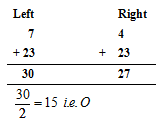Ans 17: We are required to find out the ‘middle’ letter between the 17th alphabet from left and the 6th alphabet from right. The letter, which is 6th from right is 27-6= 21st from left. Adding both the left hand side positions we get 17+21=38 and when we divide 38 by 2, the answer is 19.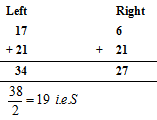Ans 18: We are required to find out the ‘middle’ letter between the 22nd alphabet from left and the 11th alphabet from right. The letter, which is 11th from right is 27-11= 16th from left. Adding both the left hand side positions we get 22+16=38 and when we divide 38 by 2, the answer is 19, and the 19th letter in the alphabet is ‘S’.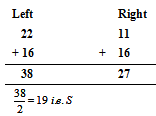Ans 19: The letter which is 3rd from right side in the alphabet series is 27-3= 24th from left side. The other alphabet is 18th from left side and when we add both the left side positions, we get  18+24=42. Dividing 42 by 2, we get 21. The 21st letter is ‘U’ is the answer.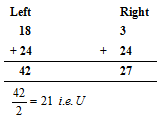Ans 20: Similarly, the answer will be 16th letter i.e. ‘P’.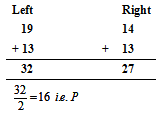CASE – 2:   When we are given only two alphabets

Sometimes we are given simply two alphabets then as we know the place values of these two alphabets, we simply add the place values of these alphabets and then divide the result by 2 to get the answer i.e. the middle position of the alphabets.

Following are the examples to find out the middle position of two alphabets in this case:

Ex 21: Which is the middle letter between H and N?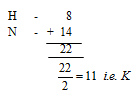Ex 22: Which is the middle letter between H and S?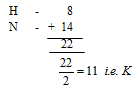CASE – 3: When we are given the position of two alphabets from R.H.S.

In this case, we simply added the place values of alphabets and then divide the result by 2 as such in case 2. But here the answer we now get that is from R.H.S, but we need the answer from L.H.S. So, according to the rule 1, we simply subtract the above answer from 27 to get the answer from L.H.S.

Ex 23:  10th  from right and 4th from right

Ex 24:   20th  from right and 4th from right

Ex 25:  18th from right and 4th from right

Ex 26:    15th from right and 16th from right

Ans 23: 10th from right and 4th from right, add both the positions, 10+4= 14 and divide it by 2= 7. The 7th letter from right is 27-7= 20th from left, so ‘T’ is the answer.

Ans 24: Adding both positions from right i.e. 20th and 4th from right, we get 24 and dividing it by 2, we get 12. The 12th letter from right side is 27-12=15th from left side of the alphabet and the said letter is ‘O’.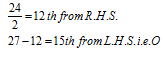Ans 25: Adding both the positions from right side, 18th and 4th , we get 18+4= 22; divide the same by 2 and we get 11. The 11th from right is 27-11= 16th from left i.e. ‘P’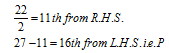Ans 26: Positions From Right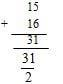Fractional value which cannot be accepted because we need the whole no. to get the answer.

RULE 4: RELATIVE POSITION BETWEEN TWO ALPHABETS:

When the relative position of two alphabets is given and one is required to find the alphabet.

The first step is to find out from which side of the alphabet series are we to find the required alphabet, from left side or the right side.

The second step is to which side are we to look further; towards left or right. If we are looking for an alphabet from left side of the series and the required one is to its right, then we add the right position to the position from left and if it is to its left, then we subtract from it.

GENERAL PATTERN: WHICH ALPHABET IS ­–TO THE LEFT/RIGHT OF––FROM LEFT / RIGHT

So for this, we can make the following as the general rule:-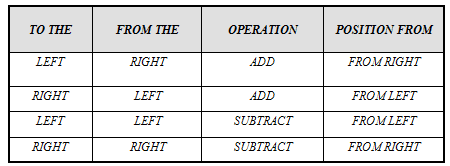Following are some of the examples:-

Ex 27: Which alphabet is 6th to left of 17th from left?

Ex 28: Which alphabet is 8th to right of 10th from left?

Ex 29: Which alphabet is 4th to left of 20th from right?

Ex 30: Which alphabet is 9th to right of 18th from right?

Ex 31: Which alphabet is 3rd to left of 22nd from right?

Ans 27: Both positions are left. So according to the above table; 17 – 6 = 11 i.e. K

Ans 28: One position is from left and other is from the right. So according to the above table;

8 + 10 = 18th from left i.e. R

Ans 29: One position is from left and other is from the right. So according to the above table;

4 + 20 = 24th from right = 3rd from left (27 – 24) i.e. C.

Ans 30: Both positions are from right. So according to the above table 18 – 9 = 9th from right = 18th from left (27 – 9) i.e. R

Ans 31: One position is from left and other is from the right. So according to the above table;

22 + 3 = 25th from right i.e. 27-25 = 2nd from left. i.e. B.

RULE 5:  ARRANGEMENT OF LETTERS IN ALPHABETICAL ORDER AND FINDING OUT THE POSITION FROM LEFT OR RIGHT

In this Rule, You must know the sequence of the alphabets by heart. So, you have to memorize the place values of alphabets so that you can arrange the letters of the given word in alphabetical order very quickly.

Following are some of the examples:-

Ex 32: If the word PENCIL is written alphabetically then which is the fourth letter from right?

Ex 33: If the word COUNTER is written alphabetically then which is the third letter from left?

Ex 34: If the word REPRESENTATION is written alphabetically then which is the second word to the left of fifth from right?

Ex 35: If the word EXAMINATION is written alphabetically then which is the third word to the left of fifth from left?

Ex 36: If the word ELECTRICITY is written alphabetically then which is the second word to the right of third from left?

Ans 32: PENCIL word when broken down can be written as CEILNP. So the fourth alphabet from right is I.

Ans 33: The said letter after it is written in alphabetical order is: CENORTU. So the third letter from left is N.

Ans 34: The said word after it is written in alphabetical order is: AEEEINNOPRRSTT. The second word to left of fifth from right is 5th + 2nd  = 7th from right i.e. O.

Ans 35: The said word after it is written in alphabetical order is: AAEIIMNNOTX. The third word to left of fifth from left is 5th – 3rd = 2nd alphabet from left i.e. A.

Ans 36: The said word after it is written in alphabetical order is: CCEEIILRTTY. The second word to right of third from left is 3rd +2nd from left = 5th from left i.e. I.

RULE 6: ARRANGEMENT OF WORDS IN ALPHABETICAL ORDER

We know that in a dictionary, the words are arranged in alphabetical order. Therefore, you must know the basics of “Dictionary Usage”. The words beginning with the same letter of English alphabet are again arranged in alphabetical order with respect to the second letter of words and so on. In order to solve such questions, First of all observe the first letter of each word. If the first letter is the same, observe the second letter and so on till you get the required arrangement of the words

Ex 37:   If the following five words are arranged in alphabetical order or as per dictionary, which of them will come at the fourth place?

(1) Nature              (2) Narrow
(3) Numerous       (4) Narrate

Ex 38:  Arrange the following words in alphabetical order or as per dictionary.(1) Plight             (2) Pledge
(3) Pugilist          (4) Pander

Ex 39:  Arrange the following words in alphabetical order or as per dictionary.

(1) Freight,             (2) Flight
(3) Feminine         (4) Friend

Ex 40:   Arrange the following words in alphabetical order or as per dictionary.
(1) Agree             (2) anonymous
(3) acrimonious      (4) antipathy

Ex 41:   Arrange the following words in alphabetical order or as per dictionary.

(1) Sundry                 (2) script
(3) season                  (4) slight

Ans 37:   When we arrange the words as per dictionary order, the positions will be as under:

At first place        ––––       Narrate

At second place       ––––       Narrow

At third place             ––––       Nature

At fourth place            ––––       Numerous

Ans 38:    (1) Pander           (2) Pledge       (3) Plight             (4) Pugilist

Ans 39:    (1) Feminine        (2) Flight         (3) freight            (4) Friend

Ans 40:    (1) Acrimonious   (2) Agree         (3) anonymous    (4) Antipathy

Ans 41:     (1) Script            (2) Season             (3) slight        (4) Sundry

Offer running on EduRev: Apply code STAYHOME200 to get INR 200 off on our premium plan EduRev Infinity!

15 docs|54 tests

,

,

,

,

,

,

,

,

,

,

,

,

,

,

,

,

,

,

,

,

,

;